# Chapter I: Basic Notions

Maurizio Rafanelli, Istituto di Analisi dei Sistemi ed Informatica – C.N.R.,

Italy

This chapter presents the basic notions regarding multidimensional (aggregate) databases by referring to different definitions given for them in the literature. It illustrates the important concepts of micro, macro, and metadata; presents a formal definition of the aggregation process, discussing the concepts of dimension and dimension hierarchies; describes the multidimensional aggregate data structure, distinguishing between simple, complex, and composite structure; illustrates the different types of null values; and discusses differences and similarities which exist between multidimensional aggregate data (generally called statistical data because they are used mainly by statisticians) and the On-Line-Analytic Processing (OLAP) of multidimensional data represented by different data cubes, also discussing the different (symmetric and non-symmetric) treatment of dimensions and measures required by OLAP and aggregate multidimensional databases. Finally it discusses a graph model and a tabular model for this kind of data, and gives a set of definitions regarding the OLAP terminology.

## INTRODUCTION

In this chapter we present the basic notions regarding multidimensional (aggregate) databases by referring to different definitions given for them in the literature. In particular, we illustrate the differences which exist between disaggregate (micro) and aggregate (macro) data structures and the importance, in this context, of metadata. The role they play in a multidimensional aggregate database is also discussed. Then, we present the process which makes it possible to obtain aggregate data (macrodata) from a very large set of raw data (microdata). In this context we introduce the concept of dimension, which will be discussed in Chapter 4, specifically the possible hierarchies which could be present in each dimension. We distinguish between two different user activities performed on these macrodata. Such activities are orthogonal to each other, and are characterized by:

1. Multidimensional Data Manipulating, i.e., the manipulation of the descriptive part of macrodata, which is metadata; this kind of activity does not change the summary types (count, percent, average, etc.) of the macrodata measure, and the fact described by the single object.

2. Data Analysis, i.e., the processing and elaboration of the summary values, which can change the summary value type (for example, from sum to percent).

Finally, we describe the different approaches used in the varying fields of application, such as the statistical environment and On-Line Analytical Processing (OLAP). Both of them use these data, but the approaches mentioned above depend on the different aspects emphasized in them. For example, most of the work they carry out is characterized by their change of use (from statistical and socio-economic type applications to the analysis of transaction-based business data).

This chapter is outlined as follows: the first section illustrates the important concepts of micro, macro, and metadata. A formal definition of the aggregation process follows, and we also briefly discuss the concepts of dimension and dimension hierarchies. We then introduce definitions of the different data structures proposed in the literature for multidimensional aggregate data (statistical object, table, cube, MAD). The different types of null values are illustrated, along with how they are treated in a multidimensional database. The next section discusses differences and similarities which exist between multidimensional aggregate data (generally called statistical data because they are used mainly by statisticians) and the On-Line-Analytic Processing (OLAP) of multidimensional data represented by different data cubes. It also discusses the different (symmetric and non-symmetric) treatment of dimensions and measures required by OLAP and aggregate multidimensional databases.

During the 1980s there was a lot of activity in the area of multidimensional aggregate databases, called statistical databases, focusing mostly on socio-economic-type applications. The fundamental data structure of a multidimensional database is what is known in the literature as an n-dimensional table (referred to by Gyssens & Lakshmanan, 1997). During the 2nd Statistical Data Base Management workshop, held in 1983 in California (see 2nd SDBM, 1983), the multidimensionality of statistical and scientific data was underlined as a/the storage structure in order to organize disk files into blocks. In particular, the majority of scientific data models had a rectangular three-dimensional grid, i.e., a parallelepiped whose individual cells were minicubes. Each rectangular three-dimensional grid was subdivided into "cubes," as proposed in Bell (1983). This paper was the first example of multidimensional representation of data by cubes. In the 1990s the On-Line Analytic Processing (OLAP) area was introduced for the analysis of transaction-based business data, such as retail store transactions (see Codd, 1993). In this area the cube metaphor was proposed again in order to underline the representation and also to support business data in a multi-dimensional space.

As discussed in more detail later in this chapter, both OLAP and multidimensional statistical databases (SDBs) deal with multidimensional datasets, and both are concerned with applications of aggregate functions over the dimensions of the data sets. Much of the work on SDBs took place in the 1980s and still continues today, as does OLAP database work, which started mainly in the 1990s.

Some of the main topics in the literature on MDDBs are: metadata, conceptual and logical data models, data structure, data selection, data manipulation, and data querying and visualization (output display).

In McCarthy (1982) the author gives a definition of metadata for large statistical databases (in this book, by statistical databases we mean a class of databases which allow the definition, manipulation, elaboration, and storing of aggregate multidimensional data, obtained by applying aggregative functions to raw data, and which are used to obtain statistical analyses):

"Metadata is data about data, that is, systematic descriptive information about data content and organization that can be retrieved, manipulated, and displayed in various ways. Metadata may be simple and unstructured, such as a typewritten narrative describing a data tape, or structured and complex, such as an active machine-readable DBMS dictionary used to control multiple databases."

Generally there are two broad classes of multidimensional statistical data and micro and macrodata, as described by Wong (1984). The former refers to SDBs containing microdata (sometimes called "elementary" or raw data), that is, records of individual entities or events, such as mortality data of individual people or population census. The latter refers to databases containing multidimensional aggregate data (MAD), often shown as statistical tables, that result from the application of aggregate functions (for example, count, sum, or average) on raw data. Examples of MAD are tables of "energy consumption" or charts of "mortality by disease." These two classes of databases differ from various points of view. For example, from a descriptive point of view, there is an intentional and an extensional level of metadata for the macrodata, unlike for the microdata; from the querying point of view, due to the different (and more complex) structure of the data, the relational algebra operators are suitably modified so as to correctly carry out queries on the relations which represent the structures mentioned above.

The distinction between metadata and data is not always a clear one. For example, consider a table of population counts by age, race, and sex. In one sense, age, race, and sex categories are data values that characterize the individuals summarized in the table. In another sense, they are metadata that serve as labels for cross-product cells of a three-dimensional structure. From a relational perspective, they may be viewed as composite keys of a single population attribute. As noted in Smith & Smith (1978), "relationship, entity, component, category, attribute, and instance are just different interpretations of the same abstract objects."

In addition to these concepts, multidimensional aggregate (statistical) databases require special types of metadata to describe statistical characteristics, and to provide information for data manipulation and analysis software. At the same time, the problem of representing conceptually aggregate data, as well as defining a suitable data structure for them arose. As it is known, a relational database consists of a large set of relations, each of them with their own relation schema, their own set of attributes (each with an instance domain), and so on. In these databases different aspects of reality can be stored, from a population census to data regarding car production of a given industry, from data on epidemiological studies to data on cars sold by a given organization, and so on. All these data are called microdata, i.e., raw data which represent different peculiarities of the reality which they describe and which refer to information of different individual entities, such as persons, objects, events, etc.

These microdata are generally stored as relations in a relational database and when an aggregation function, like a sum or a count is applied, the result is a complex data, called macrodata, which consists of a descriptive part and a summary part. The latter is called a/the summary attribute or measure, and it is characterized by the descriptive part mentioned above. This descriptive part is called metadata and its simplest definition is "data describing data." Many researchers proposed using the relational model also for this kind of data. Regarding this point, we observe that the modeling of aggregate data by means of relations has several drawbacks. In particular:

1. Each multidimensional aggregate table has its own relation scheme which corresponds to a distinct DB file at the physical level. As a result information within the database is highly fragmented and the integrated access to such information may become difficult or even unfeasible. For this reason Malvestuto (1993) proposes universal schemes to collect, in a single relation, all the multidimensional aggregate tables regarding the same population of units of observation, i.e., obtained from the same set of microdata. Similarly, Sato (1991) introduces conceptual files to unify all the aggregate data corresponding to the same abstract concept, i.e., which describe the same phenomenon.

2. As already stressed in Chan & Shoshani (1981) and, successively, in many other papers, conventional database organizations are not adequate to exploit the regularity induced by the cross-product of the category attribute domains and therefore this results in large redundancies. The introduction of universal schemes and indexing techniques increases such redundancies further.

3. The operators of the relational algebra are inadequate at manipulating aggregate data correctly. Let us consider for example a relation scheme R (Year, Nation, Sex, Population, AvgIncome), where the underlined words represent the category attributes or descriptive parameters which form a key of R, and Population and AvgIncome represent the measures of the fact studied, classified by year, nation, and sex. If only the population and average income statistics, classified by year and nation, are required (such values are known as marginal values, as defined in Malvestuto, Moscarini, & Rafanelli, 1991), one has to "remove" the (category) attribute sex. However, a projection would obviously yield an incorrect value. On the other hand, the operation where a simple projection would work correctly is the removal of the (summary) attribute AvgIncome from the same scheme R. Consequently, several extensions of the relational model have been proposed by Ghosh (1985), who sometimes distinguishes category and summary attributes in generalized relations, such as in Su (1983), or by allowing set-valued relations and introducing new specific operators, as in Ozsoyoglu, Ozsoyoglu, & Mata (1985), and in Ozsoyoglu, Ozsoyoglu, & Matos (1987). However, as noted by different researchers, multidimensional aggregate data is fairly complex. It needs to support semantics, operations, and physical structures of multidimensional space, as well as classification structures. Supporting classification structures implies the storage and management of all the metadata of the category values, and their hierarchical associations. For this reason a different approach was studied. It was based on different data structures and operators, which were able to consider the complexity of the data.

### The Aggregation Process

In this section we present a formal description of the aggregation process, in order to achieve a more precise modeling of aggregate data. We will define these data from both a conceptual and a logical point of view.

The main difference between them is that in the case of conceptual data, which we will call multidimensional aggregate data (MAD), we do not consider their physical storage, while in the case of logical data, which we will call the multidimensional aggregate data structure (MADS), we refer explicitly to their physical storage. In the following we will give their formal definitions and show that this structure corresponds exactly with the aggregation process.

Before speaking about the aggregation process, we describe the concept of the aggregation function as presented in Klug (1982). This concept is quite simple. An aggregation function takes a set of tuples (a relation) as an argument and produces a single simple value (usually a number) as a result. Many relational query languages require that aggregate functions are able to accept arguments with duplicates. For example, to sum the salaries in an employee relation, the relation would be projected on the salary column, duplicates would be retained, and the projection would be sent to the sum function. Besides being unnecessary, as we will see, using the notion of "duplicates" has a number of disadvantages. For example, a disadvantage is the following: the usual algebraic identities for relational algebra fail to hold when duplicates must be retained. The author gives one example of this. Consider the following two "relations" with duplicates: Intersection should be related to join and projection by the equation,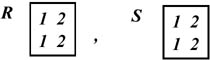Intersection should be related to join and projection by the equation,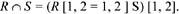The right-hand part evaluates the relation,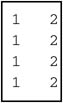Now, intersection should also satisfy the property,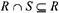Clearly, this is impossible.

The solution proposed by the author is quite simple. Instead of providing one sum function (or average, max, etc.), he provides a parameterized family of sum functions: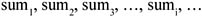The function sumi sums the numbers in the ith column of its input. Now there is no need for the notion of "duplicates." For example, to determine the sum of salaries (column 3) in the relation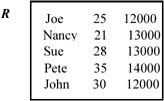we would write sum3(R).

Formally, we hypothesize a countable set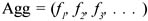of aggregate functions. Each fi Agg is a function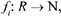where R is the set of all relations. Thus, an aggregate function, given a set of tuples, produces a single number as its value.

Most of the authors describe multidimensional aggregate data as a mapping from the domains of the category attributes (independent variable) to the (numerical) domains of the summary attributes (dependent variable). Each category attribute often represents a level of a hierarchy present in that dimension of that MAD. Since the independent variable ranges over an n-dimensional space (the space of the n-tuples of category attribute instances), in Shoshani & Wong (1985) the concept of multidimensionality of aggregate data was introduced. Consequently, many of the authors mentioned above proposed modeling aggregate data by means of particular relations where the category attributes form the key. In other words, the category attributes C and the summary attributes S are the building blocks of a relation scheme R = C, S, where the functional dependency C S holds. Such data can assume different graphical representations: generalized relations (as proposed in Su, 1983), statistical tables, histograms, pie-charts, graphics, cubes, etc. For the sake of simplicity, unless differently specified, we will consider a table as the base structure. A multidimensional aggregate table can be represented by a relation r over R such that each tuple in r corresponds to an entry in the table.

Now we give some definitions useful in describing the aggregation process.

Let Θ be the database universe, i.e., the set of all the relations which form the very large relational database in which raw data (microdata) are stored (for example, a census made in a given year and in a given country, data on production of a given industry in the last 20 years, and so on).

Let R be the subset of Θ relative to all the relations used in the definition of the multidimensional aggregate (macro) database and which, therefore, refers to all the phenomena studied. Note that each phenomenon consists of one or more facts which are the physical objects stored in the database. For example, a phenomenon can be "Production of cars" and the set of facts which refer to this phenomenon could be: "Production of cars in Italy classified by year of production, manufacturer, and model"; "Production of cars in Italy classified by year of production, builder, and color"; "Production of cars in France classified by year of production, color, and model"; and so on.

Let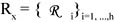be the set of all the relations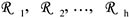(each of them with attributes different in number and names), which refer to the x-th phenomenon. Let A11, A12, , A1k1 be the set of attributes of the relation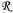1, where the apex refers to the index which characterizes the considered relation, k1 is the number of attributes of this relation (i.e., its cardinality), each of which has a definition domain Δi1, Δi2, , Δik1, and likewise for the other relations. Note that, in general, not all the attributes are used in the definition of one fact.

In order to clarify how the subsets of R to be aggregated are characterized, let us analyze the well-known concept of the category attribute of a multidimensional aggregate database. A category attribute is the result of an abstraction on one or more attributes of the microdata; analogously its instances are the result of an abstraction on the (numerical, Boolean, string, etc.) values actually associated with the single microdata. Abstraction plays an important role in the aggregation process, because in general many attributes with different structures and names may correspond to the same category attribute. In order to be aggregated, the microdata must "talk about the same thing," but this by no means implies that the way of doing it is the same in all microdata. Thus, the same category attribute "town" of a given statistical object may correspond to a 30-character string field named "TOWN" in a group of microdata, to a 40-character string field named "city" in a second group of microdata, and even to a numerical field named "town_code" in another group.

Let Ω be the set of all the attributes which appear in R, and let Ax Ω be the generic attribute of this set.

Definition 1 Let R be the set of all the relations used in the definition of a multidimensional aggregate database, let Ax Ω be a generic attribute of this database, and let axy be one of its instances (with y = 1,, k, where k is the cardinality of the definition domain of Ax). The logical predicate (Ax = axy), defined on the microdata of R, is called base predicate.

For example, if Ax is the generic attribute "Year" and if its instance domain is <1990, 1991, 1992, 1993, 1994, 1995>, the base predicate Ax = ax1 is Year = 1990.

Definition 2 The base set of the base predicate (Ax = axy) is the subset of Θ consisting of all microdata which satisfy the base predicate. In the following such a subset will be denoted by BAx = axy.

Let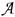be the subset of all the attributes of Ω that will become descriptive (or category) attributes or measures of all the MAD which will form the multidimensional aggregate database at the end of the aggregation process. Thenis the set of all and only the attributes which describe all the facts which appear in the multidimensional aggregate database. Many of these attributes appear in different relations of R. Different attributes can contribute to form one hierarchy. Different hierarchies can belong to the same dimension, on the condition that pairs of hierarchies have at least one attribute in common. Note that parallel hierarchies, called specialization hierarchies, can exist.

For example, country state city can have, as a specialization hierarchy, country_of_residence state_of_residence city_of_residence. Moreover, other attributes, which do not appear in, can complete the hierarchies mentioned above (on the condition that the relationship between them and the other attributes of the same hierarchy is defined). We call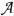the set of these last attributes plus the attributes of.

For example, supposing, in the microdatabase, we have the attributes city, zone, state, region, and country which are all linked in this order by a part-of relationship. Also supposing the aggregation process created a set of fact tables in the multidimensional aggregate database which only has the category attributes city, state, and country forming the above-mentioned hierarchy of the dimension "space." Then, setconsists of the attributes "city, state, and country," while setconsists of the attributes "city, zone, state, region, and country."

We call the latter hierarchies primitive hierarchies because all the hierarchies which refer to one of them are included in it. Analogously, we call the dimension which includes all its primitive hierarchies the primitive dimension.

Let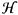be the set of all the hierarchies (including the specialized hierarchies) defined in. Let D be the set of all the dimensions defined in(which can consist of different hierarchies).

Note that the users often give the name of a dimension to descriptive variables of a MAD which are, in reality, levels of a hierarchy relative to this dimension. For example, they say "Production of Cars, classified by the dimensions model, year of production, and manufacturer," where the dimension year of production is, in reality, the level year of production of the (specialized) hierarchy "year of production month of production day of production" of the dimension "time." In fact, this last is a conceptual structure which can have different category attributes referring to different hierarchies of the same dimension in the same MAD. As another example, we can consider a given MAD which represents the fact "Work situation in USA" classified by "birth state" and by "state of residence," which are two specialization hierarchies of the same hierarchy in the same (primitive) dimension, but which define two different dimensions in this MAD.

Let Δ be the set of all the definition domains (i.e., of all the instances) of the attributes of, and let Δ be the set of all the definition domains of the attributes ofwhich also include all the possible instances that each attribute can assume (therefore, also including the instances not present in the relations of Θ). We call these definition domains "primitive domains." This means that all the attributes (and all the relative instances) which appear in the multidimensional aggregate database are part ofand Δ respectively.

Category attributes are not the only metadata of multidimensional aggregate data: several other properties may provide a semantic description of the summary data. Among them we consider, in particular the following:

• the aggregation type, which is the function type applied to microdata (e.g., count, sum, average, etc.) to obtain the macrodata (i.e., a MAD, see Tansel, 1987; Rafanelli & Ricci, 1993) and which defines the summary type of the measure. This property must always be specified;

• the data type, which is the type of summary attribute (e.g., real, integer, non-negative real, non-negative integer) (see Malvestuto, 1993);

• the fact Fj described by the multidimensional aggregate table considered (e.g., production, population, income, life expectancy) (see Bezenchek, Massari, & Rafanelli, 1994);

• other properties may be missing, for example "data source" (which may be unknown), "unit of measure," and "unit of count," as defined in the following.

Let Γ be the set of the functional dependencies which are possibly present in the multidimensional aggregate database and which, therefore, exist among groups of attributes. Functional dependency is another important concept which it is necessary to consider in order to manipulate the MADS of the multidimensional aggregate database correctly. For example, if one fact stored in the MDDB is "Car production in Italy," described by year of production, model, color, etc., and another fact stored is "Car production in France," described by cylinder, color, etc., after having defined the functional dependency "year of production, model cylinder," we can join the two MADS by aggregating the first MADS to "cylinder." Obviously, the result will be at a minor level of descriptive granularity.

Given a phenomenon x and given the set of relations Rx R, we consider the subset of Rx formed only by the relations involved in the building of the fact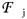. We call this subset an aggregation relation, and denote it by Rjx, where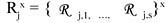. Every fact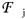has its own descriptive space formed by s category attributes (where s is the cardinality of the j-th fact), which are a subset of all the attributes in the relations Rjx. We denote the set of the above-mentioned s category attributes by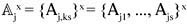We call the relation Bjx, formed by these attributes, a base relation of the fact.

The measure values are the result of the aggregation process, i.e., of the application of the aggregation function to the base relation of the fact. The fact obtained by this aggregation process is called base fact, because its representation cannot even be disaggregated (i.e., only more aggregate views can be obtained). Each fact consists of a set of materialized views, obtained by applying different operators of aggregation (roll-up, group-by), or of reduction of the definition domains of its category attributes (dice). For a discussion on operators, see Chapter 5. This set of materialized views defines the lattice of this fact. The source of this lattice is formed by the total of all the summary category instances of the base fact (called grand total), and the sink formed by all the summary category instances at the lowest level of disaggregation.

Let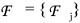be the set of all the fact names described by the MAD of the multidimensional aggregate database.

Let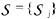be the set of all the subjects described in the facts, in other words, the "what is" of the summary attributes (cars, people, fruit, workers, dollars, etc.).

Let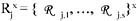be the subset of the relations in the microdatabase which are involved in the x-th fact. Let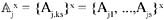be the set of attributes of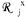which are the only ones considered in the building of this MAD.

Definition 3 Let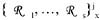be the set of all the relations involved in the building of the generic j-th fact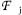of the x-th phenomenon Px. These relations are included in Rx and have all the category attributes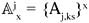of the fact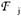simultaneously present in it (and possibly other attributes which are not used in this aggregation process). ‘j’ characterizes the different category attributes of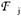. We call the relation (in non-normal form) formed by all the tuples of the previous set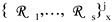aggregation relation Rjx of the j-th MAD which describes the fact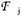.

For example, let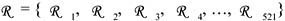be the set of all the relations which refer to the set of facts F studied in the multidimensional aggregate database. Let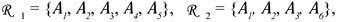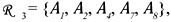, and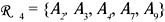be the set of relations which refer to the j-th fact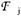described, for example, by the category attributes A1, A2, A4. Let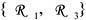be the set of relations which have the set of all the attributes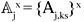(with ks = 1,2,4) of the fact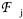simultaneously present. The descriptive space of the MAD which describes the fact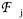, the relations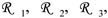, and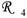which refer to the fact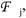, and the aggregation relation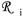of a MAD which describes the fact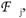, are shown in Figure 1.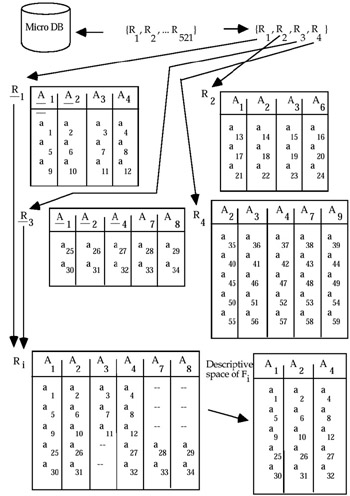Figure 1: Phases of the Aggregation Process

Definition 4 The classification set ζAx of the category attribute Ax with the domain ΔAx is the set whose elements are the base sets defined on Θ by Ax: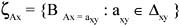For example, if year = { 1991, 1992, 1993 }, then: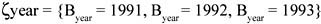Definition 5 By υBAx we denote the union of all base sets defined on Θ by the instances of the category attribute Ax: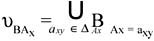and we call the set {υAx} union set of Ax.

For example, if the attribute year has the same domain indicated above, then: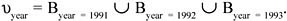Definition 6 We call aggregation schema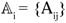of the i-th MAD, with the aggregation relation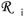which describes the fact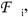the set of all the names of the category attributes Aij of the fact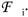This set defines the dimensions of the MAD. The number of these category attributes defines the cardinality of the MAD.

Definition 7 The base relation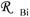of the i-th MAD (with its predefined descriptive space), which describes fact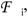, is the subset of the aggregation relation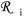of this fact which has all and only the descriptive attributes ABij of the fact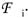For example, the base relation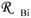of the i-th MAD of the previous example is its descriptive space formed by the attributes Aij (with j = 1,2,4) of the i-th fact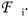Definition 8 The base schema Aij of the i-th MAD is the tuple of the base relation attribute names of the MAD.

Now we can give a more precise definition of the base set.

Definition 9 The base set BAx = axy of the base predicate Ax = axy relative to the base relation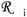of the i-th MAD is the subset of the base relation tuples which satisfy the base predicate.

Now we can describe the aggregation process, starting from a relational database of raw data Θ. Supposing, for example, we refer to "Data on production in Italy." This production refers to transportation, food, building trade, clothing trade, etc.

The first step in building an aggregate database is to define set R of all possible microdata relations to which the aggregation process must be applied, and, within it, to choose the set Rx of relations which refer to a given phenomenon Px. Suppose that R x refers to the phenomenon Px = "Data on transportation" and that the relations involved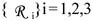are Car (make, model, cubic capacity, color, air-conditioning, automatic gears, number of airbags, month-year of production), Sale (make, model, cubic capacity, arrival date, sale date, name of the seller, branch office city, zone of sale), and Manufactures (manufacturer's name, sex, age, region of residence, make, model, cubic capacity, color).

Then, having defined set Ω of all the attributes which appear in R (i.e., make, model, cubic capacity, color, air-conditioning, automatic gear, number of airbag, month-year of production, arrival date, sale date, seller name, city of the branch, sale zone, buyer's name, sex, age, region of residence), the second step consists of choosing attributes which will become descriptive (or category) attributes in the multidimensional aggregate database, that is, set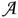(in this case, for example, {model, cubic capacity, color, month-year of production, arrival date, sale date, city of the branch, sale zone, sex, age, region of residence}.) In this step we also define the possible functional dependencies, as well as the attributes which possibly complete the hierarchies, and the specialization hierarchies. For example, in this case, a functional dependency is (model, month-year of production cubic capacity).

The third step consists of recognizing all the attributes which belong to the same dimension, and the hierarchies (and their specializations) within this dimension, i.e., sets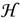and D. With reference to the example mentioned above, we have different hierarchies (time, space, etc.), which form set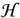. In this step, all the attributes which do not appear explicitly but which complete a given hierarchy, as well as the possible specialization hierarchies (explained in Chapter 4), have to be inserted into it. In our example, we define the first hierarchy of the dimension "space," i.e., region province city, then the connected specialization hierarchies, i.e., region (of residence) province (of residence) city (of residence), and region (of branch office) province (of branch office) city (of branch office). Note that the instances of specialization attributes are exactly the same instances as the reference attributes, even if the semantics are different. At this point we define the second hierarchy of the dimension "space," i.e., zone (of sale) city (of sale). Note that the two hierarchies have (at least) one attribute in common (in this case, city). In this step the complete definition domains of all the above-mentioned attributes (then the primitive domains) will also be defined, i.e., set Δ*.

At this point, the part of the multidimensional aggregate database which refers to the phenomenon "Data on transportation" can be built repeatedly by performing the following steps.

The fourth step is selection of the subset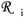of relations which are involved in the i-th fact, and of the set Aikn of attributes ofwhich are the only ones considered in the building of the MAD. In this way we have defined the general characteristics of this object. At the end of the aggregation process, all the sets Aj of attributes will form the descriptive space of the multidimensional aggregate database. In this step we also define the subsets in D and in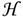which characterize respectively the dimensions and the possible hierarchies in each dimension of the fact. Therefore, a hierarchy represents the same dimension at different granularity levels.

One of the innovative features of multidimensional aggregate data has been the introduction of a third type of attribute, namely the implicit category attribute, which can considerably enlarge and enhance the manipulation capabilities of an aggregate database. In fact, if among the attributes which define the dimensions of a MAD, one or more of them have a definition domain which consists of only one instance, we transform each of them into part of the "fact name" and call it an implicit attribute or an implicit dimension (because it does not explicitly appear in the MAD dimensions). Note that also implicit attributes contribute to MAD cardinality (i.e., the number of dimensions present in this MAD).

The fifth step involves application of the aggregation function to the attributes of the relations in A. The result of this operation is the numeric data which represents the measure carried out on microdata, called simply measure (or summary attribute). Depending on the type of function applied, the parameters which characterize each fact have to be defined, i.e.:

• summary type—defined by the aggregate function type applied to microdata (a count, a sum, etc.);

• the count unit—suppose that the result instances are 100, 213, 47, etc. and suppose also that the subject is "fruit"; the count unit defines if, for example, 100 really means 100 (100 x 1), or 100,000 (100 x 1,000) or 100,000,000 (100 x 100,000), and so on;

• measure unit—in the previous example, the number could be "number of pieces of fruit," or "kilograms," or "tons," etc.;

• data source (this information is not always available).

In general, the aggregation function applied is initially a count or a sum. Subsequent aggregations can be obtained by applying algebraic operations and statistical-mathematic operations to these aggregate data, for example, to obtain averages, percentages, maxims, minimums, index functions, and so on.

The sixth step is definition of the fact name (name of the MAD), for example Production of vegetables, Sale of cars, Number of births in a given state, Incidence of a given disease in a fixed country, etc. At the end of the aggregation process, all the names of the facts defined will form the set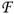.

The seventh step then is definition of the subject described in the fact, by choosing it from among the attributes of the relations in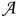(for example, cars). At the end of the aggregation process, all the subjects described in the facts will form set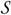.

The eighth step is definition of possible Notes which characterize possible anomalies of the MAD (this step can be lacking). For example, if car production (name of the fact described by the MAD) is described by year of production, region, and model, we could have the summary data for the years 1985, 1990, 1995, and 2000 for all the regions, apart from the region Piedemont, where car production refers to the years 1986, 1991, 1996, and 2001. In the MAD these last data are classified as if they were relative to the years 1985, 1990, 1995, and 2000, but, with a suitable Note, we would know the real years.

When we apply the aggregation process, all the MADs produced are conceptual structures, in the sense that, for each of them, we have not yet defined how to store them in the database, or any order among the attributes of a fact or among the domain instances of each attribute. To store them in the multidimensional aggregate database, we have to define these orders.

The result of this further step (the ninth step) is the definition, for each MAD, of a corresponding logical structure, called Multidimensional Aggregate Data Structure (MADS). In it each instance of the measured data is characterized exactly by a tuple, whose elements are defined by one instance for each dimension (category attribute) of the MAD.

Definition 10 An aggregation process is formally described by the six-tuple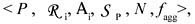where:
P is a fact name which identifies a fact universe R through a mapping φ; this mapping is defined from the fact set F to the powerset of Θ.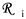is a set of relations involved in the building of the i-th MAD.

Ap is a set of the descriptive (category) attributes {Ap,1, Ap,2,, Ap,k} of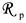(with its own instance domains Δ(Ap,j), with j = 1,, k) on which to apply an aggregation function.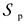is the subject which characterizes the measure of the MAD.

N is a numerical domain.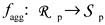is an aggregation function (initially, in general, a sum or a count).

A MAD is, therefore, a concise representation of the aggregation process result.

Definition 11 Let H and K be two sets whose elements are sets; we define the cross intersection of H and K, and denote it by H K, as the set of all the possible intersections between each element in H and each element in K: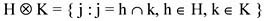In order to clarify the meaning of these definitions, let us consider a simple MADS (represented as a table), shown in Figure 2, whose fact studied is cereal production in some European countries during the period 1980–81. In it the summary type is sum, the count unit is thousands, the measure unit is ton, and the data source of the MADS is not specified.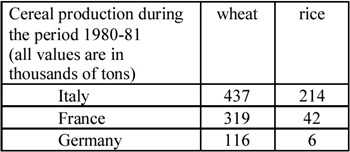The described fact is "cereal production." The category attributes "country" and "cereal" (directly obtainable from the rows and columns of the table) are not sufficient to fully characterize the aggregation sets. For instance, the cross intersection of the base set Bcountry = Italy of the base predicate "country = Italy" with the base set Bcereal = wheat of the base predicate "cereal = wheat" determines the set of all microdata regarding wheat production in Italy and such a set also includes data relative to the periods before 1980 and after 1981.

To characterize the base relation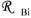of the i-th MAD (with its predefined descriptive space) correctly, which describes fact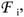we must introduce a third category attribute "years," implicitly present in the textual description of the table. Then, into the base relation (and, therefore, into the base schema) of this fact we have to insert all the category attributes which do not appear explicitly in the relation because they always assume the same (unique) value (in our case, the category attribute "years" with its definition domains formed by the set-value {1980, 1981}). We define the attributes like "years" as implicit, because they do not explicitly express a classification in the MAD, but are necessary for a correct definition of the base relations. The use of implicit attributes takes account of the full dimensionality of the MAD and as a consequence makes their manipulation more effective.Multidimensional Databases: Problems and Solutions
ISBN: 1591400538
EAN: 2147483647
Year: 2003
Pages: 150